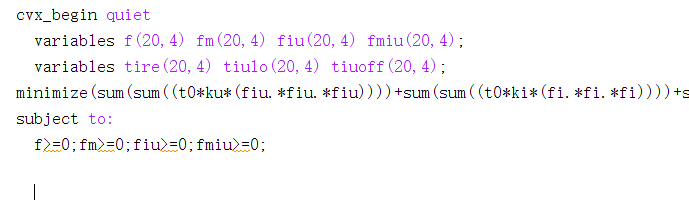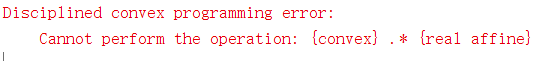# Why is the error reported {convex} .* {real affine}

The goal is the sum of each element in the matrix to the third power, and I put a non-negative constraint on the constraint, and the cubed of an unknown quantity is convex if the unknown quantity is greater than zero, so why is this still an errorBecause you didn’t follow CVX’s rules.

Use `pow_pos` or `pow_p`, with p = 3.

1 Like

Thank you very much. Could you tell me how to write such a goal? I have tried many ways, but they are all wrong
t and c are both constant，u is a variable of 2*1 dimensions
Thank you very much

You need to use `square_pos` when squaring `norm`.

1 Like

Thank you very much for your warm reply，I’ve got a new problem
This error occurs when I run the following code

Disciplined convex programming error:
Illegal operation: pow_p( {convex}, {-1} )

``````for i=1:I
for k=2:N
sum21(k,i)=0;sum22(k,i)=0;
for n=2:k
G2=B*log2(1+Pi(n,i)*inv_pos(H^2+2*(u(:,n)-si(:,i))'*u(:,n)));
sum21(k,i)= sum21(k,i)+tiulo(n,i)*G2;
L2=t0*fiu(n,i)/ci(i);
sum22(k,i)=sum22(k,i)+L2;
end
sum21(k,i)<=sum22(k,i);
end
end
``````

There’s only one 2 N variable u, and the other parameters are known，G2=Blog2(1+Pi(n,i)inv_pos(H^2+2(u(:,n)-si(:,i))’*u(:,n))); is error

The argument of `inv_pos` needs to be concave. But it is not; hence the error.

Have you proven the optimization problem is convex?

I refer to a paper, which also uses CVX to solve a problem similar to mine, and proves that G2 is convex for u(n), but I don’t know why I made a mistake in writing the code